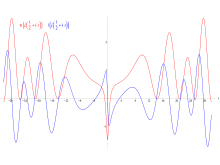Latest News
Sunday, 15 November 2015

# Millennium Prize Problem Solved By A Nigerian Scholar

The Millennium Prize Problems are seven problems in mathematics that were stated by the Clay Mathematics Institute in 2000. As of October 2015, six of the problems remain unsolved . A correct solution to any of the problems results in a US \$1,000,000 prize (sometimes called a Millennium Prize) being awarded by the institute. The Poincaré conjecture was solved by Grigori Perelman, but he declined the award in 2010.

Out of the six remaining unsolved problems, the Riemann hypothesis has recently been solved by a Nigerian scholar.In mathematics, the Riemann hypothesis, proposed by Bernhard Riemann ( 1859), is a conjecture that the non-trivial zeros of the Riemann zeta function all have real part
1/2. The name is also used for some closely related analogues, such as the Riemann hypothesis for curves over finite fields .

The Riemann zeta function ζ( s) is a function whose argument "s" may be any complex number other than 1, and whose values are also complex. It has zeros at the negative even integers; that is, ζ( s) = 0 when "s" is one of −2, −4, −6, and so on. These are called its trivial zeros. However, the negative even integers are not the only values for which the zeta function is zero. The other ones are called non-trivial zeros.

The Riemann hypothesis is concerned with the locations of these non-trivial zeros, and states that: The real part of every non-trivial zero of the Riemann zeta function is 1/2.

Dr. Opeyemi Enoch is reported to have solved the problem that remains unsolved for the past 156 years.

With this breakthrough, Dr Enoch, who teaches at the Federal University, Oye Ekiti (FUOYE), has become the fourth egghead to resolve one of the seven Millennium Problems in Mathematics.

The Kogi State-born mathematician had, before now, worked on mathematical models and structures for generating electricity from sound, thunder and Oceanic bodies.

A statement in Ado Ekiti yesterday said Dr Enoch presentation of the Proof on November 11, 2015 during the International Conference on Mathematics and Computer Science in Vienna, Austria becomes more symbolic coming on the exact day and month 156 years after the problem was delivered by a German Mathematician in 1859.

The Riemann Zeta Hypothesis is one of the seven Millennium problems set forth by the Clay Mathematics Institute with a million Dollar reward for each solved problem for the past 16 years.

According to the statement, “Dr Enoch first investigated and then established the claims of Riemann. He went on to consider and to correct the misconceptions that were communicated by Mathematicians in the past generations, thus paving way for his solutions and proofs to be established.

"He also showed how other problems of this kind can be formulated and obtained the matrix that Hilbert and Poly predicted will give these undiscovered solutions. He revealed how these solutions are applicable in cryptography, quantum information science and in quantum computers", it stated.

You can be \$1,000,000 richer if and only if you will pick one of the remaining unsolved problems to solve and give us a generally accepted solution with proof. One of it is the Birch and Swinnerton-Dyer conjecture.

Joseph Etim

Joseph Etim (B. Tech) is a blogger, mobile web developer and a tutor with a zeal for positive effects.

Website: Pepetimsblog